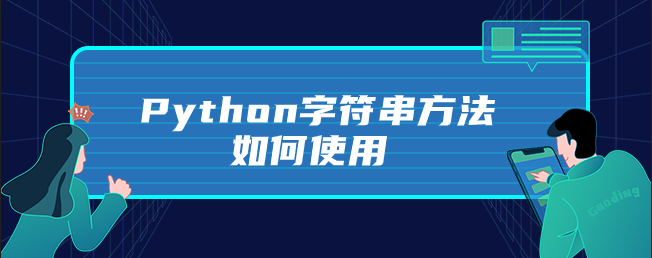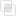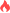Python字符串方法如何使用

Python字符串2021-06-01 17:11:031281浏览 · 0收藏 · 0评论1、find方法可以在一个较长的字符串中查找子串，他返回子串所在位置的最左端索引，如果没有找到则返回-1

```a = 'abcdefghijk'
print(a.find('abc'))                                  #the result ： 0
print(a.find('abc',10,100))                           #the result ： 11  指定查找的起始和结束查找位置```

2、 join方法是非常重要的字符串方法，是split方法的逆方法，用来连接序列中的元素，并且需要被连接的元素都必须是字符串。

```a = ['1','2','3']
print('+'.join(a))                                    #the result ： 1+2+3```

3、split方法,是一个非常重要的字符串，它是join的逆方法，用来将字符串分割成序列

`print('1+2+3+4'.split('+'))                          #the result ： ['1', '2', '3', '4']`

4、strip 方法返回去除首位空格（不包括内部）的字符串

`print("   test   test    ".strip())                  #the result ：“test   test”`

5、 replace方法返回某字符串所有匹配项均被替换之后得到字符串

`print("This is a test".replace('is','is_test'))     #the result ： This_test is_test a test`python字符串的概念及拼接python字符串需要注意的语法问题python字符串的翻转实现的两种方法python字符串的拆分与合并Python字符串有哪几种表示形式Python字符串驻留的原理探究python字符串有几种常见方法python字符串常用方法有哪些python字符串如何取值python字符串格式化的方法整理python字符串如何简单运算python字符串索引的用法python字符串中有哪些方法python异常中else的使用885

python捕获多个异常的规则1181

python ReLU函数是什么1234